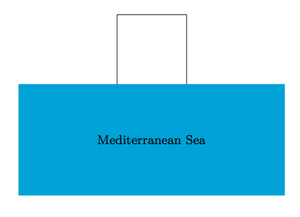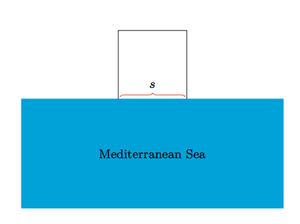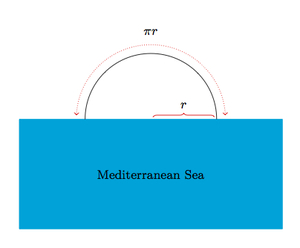# Dido and the Foundation of Carthage

Alignments to Content Standards: F-LE.A

According to ancient legend, Dido made a deal with a local ruler to obtain as much land as she could cover with an oxhide. Dido interpreted ''cover'' in an imaginative way and the land she obtained became the site of the important ancient city of Carthage (located in modern day Tunisia).

Suppose that the oxhide is an 8 foot by 8 foot square. One side of the city is made by the coastline which we will model by a straight line. Dido cut the oxhide into very narrow strips and laid them out to make the rest of the city's boundary.

1. If Dido cuts the oxhide into $k$ strips, each 8 feet long, what area will she be able to enclose if she makes a square piece of land as pictured below?2. If Dido cuts the oxhide into $k$ strips, each 8 feet long, what area will she be able to enclose if she makes a semicircular piece of land?
3. If Dido wishes to enclose a semicircle of radius 10 miles, into how many strips will she need to cut the oxhide?

## IM Commentary

The goal of this task is to interpret the mathematics behind a famous story from ancient mythology, giving rise to linear and quadratic expressions which model the story. Here is a summary of the part of Dido's story presented here:

Eventually Dido and her followers arrived on the coast of North Africa where Dido asked the Berber king Iarbas for a small bit of land for a temporary refuge until she could continue her journeying, only as much land as could be encompassed by an oxhide. They agreed.

This excerpt is taken from http://en.wikipedia.org/wiki/Dido_(Queen_of_Carthage). More can be found in Virgil's Aeneid.

The mathematics behind Dido's problem is deep and rich. In modern terms, it is called the ''isoperimetric problem'' and its rigorous mathematical solution requires showing the ''isoperimetric inequality''. More about this can be found at http://en.wikipedia.org/wiki/Isoperimetric_inequality.

## Solution

In the solution we will treat the oxhide as if it does not have width: this is a reasonable assumption when $k$ is large as it must be if Dido wishes to claim a large plot of land. This is important for the square plot of land as otherwise we have to pay close attention to what happens at the corners. It is also important for the semi-circular plot of land because it is not technically possible to make a semi-circle out of strips with the same width (because the inner circumference is not equal to the outer circumference).

1. Below is a picture showing the sea and the square plot of land, with side length $s$ that Dido encloses with the $k$ strips of oxhide.Since Dido has $8k$ feet of oxhide and needs to make three of the four sides of the square, she can construct a square with side length $s = \frac{8k}{3}$ feet (as long as $k$ is divisible by $3$): this means that the side length of the square made with $k$ strips of oxhide is a linear function of $k$, the number of strips. The area that she will enclose is $$\frac{64k^2}{9}$$ square feet. Unlike the side length $s$ which was modeled by a linear function, the area is modeled by a quadratic expression of $k$.

2. Below is a picture showing the sea and the semicircular plot of land that Dido encloses with the $k$ strips of oxhide.Each strip of oxhide is 8 feet long so Dido's semicircle has a perimeter of $8k$ feet. Letting $d$ denote the diameter of the circle, the circumference of the circle is $\pi \times d$. So the perimeter of the semicircle is $\pi \times r$. We have $$8k = \pi \times r$$ so $r = \frac{8k}{\pi}$: the radius of the semi-circular region is modeled by a linear function of $k$. The area of the semicircle is $\frac{\pi r^2}{2}$ so with $k$ strips of oxhide Dido is able to enclose an area of $$\frac{\pi}{2} \times \left(\frac{8k}{\pi}\right)^2 = \frac{32k^2}{\pi}$$ square feet. Comparing with part (a), Note that $\frac{64}{9} \lt \frac{32}{\pi}$ so the semicircle encloses a greater area than the square. As in part (a), the area Dido can enclose is a quadratic expression of $k$.

3. If $r$ is 10 miles then the semicircle has a perimeter of $10\pi$ miles or $52,800\pi$ feet. If we choose $k$ so that $$8k = 52,800\pi$$ we find that $k = 6,600\pi$. Unfortunately, $k$ needs to be a whole number but $\pi$ is an irrational number and so 6,600$\pi$ is also irrational. So Dido needs to cut the oxhide into a whole number of equal strips larger than 6,600$\pi$. To get a sense of how thin these pieces would need to be, we can observe that there are 304.8 millimeters in a foot and 2,438.4 millimeters in 8 feet. So Dido would need to slice the oxhide into pieces that are about 1/10 millimeter in width! This is not realistic but it is appropriate for a myth.